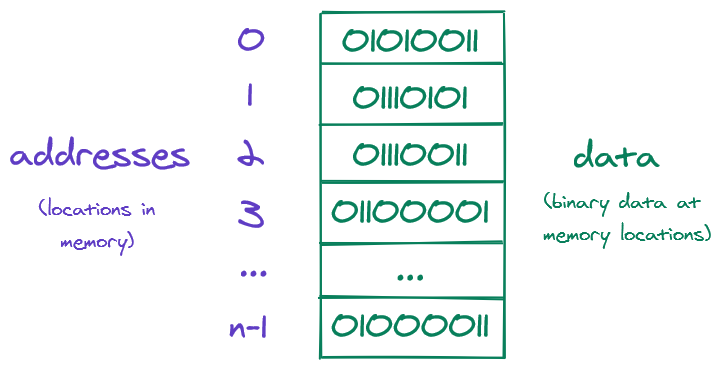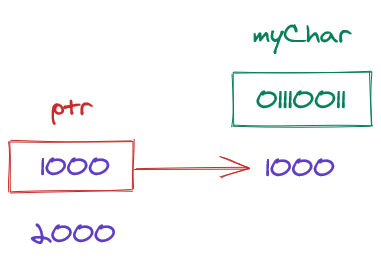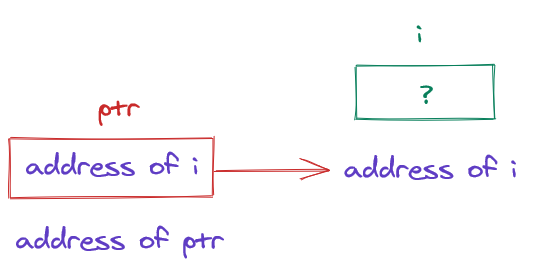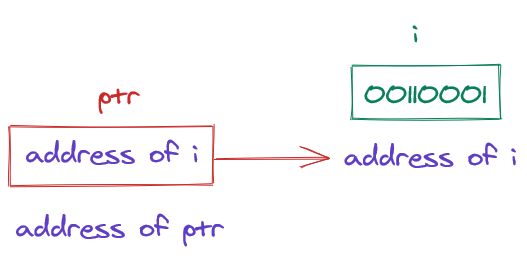Pointers in C

# Pointers in C

2020-04-11T23:46:37.893Z

Sources:

In most modern computers, main memory is divided into bytes, each capable of storing eight bits of information. Each byte has a unique address, distinguishing it from other bytes in memory. Each variable in a program occupies one or more bytes of memory.Memory address to n-1 and binary data

A memory address can be stored in a pointer variable—variables hold values just as pointers hold addresses. When we store the address of a variable `i` in the pointer variable `p`, we say that `p` "points to" `i`. A pointer stores an address, more specifically, a pointer stores the base address or initial address of a value in memory—the memory location where the first byte of the value is stored. A pointer is an arrow to the memory address where the value starts.Pointer `ptr`, located at memory address `2000`, contains (i.e. "points to") memory address `1000`, where binary data `011100111` (equivalent to character `s`) and variable `myChar` are located.

## Declaring a pointer

To create a pointer:

``````int *ptr;
``````

You declare the data type for the type of the value that the pointer may point to, then declare the pointer name preceded by an asterisk `*`, which makes it clear that this is a pointer declaration, not an ordinary variable declaration. The example above creates a pointer `ptr` capable of pointing to variables of type `int`. The asterisk `*` here simply tells the compiler that `p` is a pointer.

A pointer is also a variable, so here non-pointer variables are called "ordinary variables" to prevent confusion.

Examples of pointers to various types of ordinary variables:

``````int *ptr1; // ptr1 may only point to integers
float *ptr2; // ptr2 may only point to floats
char *ptr3; // ptr3 may only point to chars
``````

Joint declaration of ordinary variable and pointer:

``````int myChar, *ptr;
``````

## Initializing a pointer

To store a memory address in a pointer:

``````// declare ordinary variable `i`, and assign value `5` to it
int i = 5;

// declare int pointer `ptr`, and assign __address of `i`__ to it
int *ptr = &i;
``````

Or jointly declared:

``````int i = 5, *ptr = &i;
``````

As long as pointer `ptr` points to ordinary variable `i`, `*ptr` is an alias for `i`, meaning that `*ptr` has the same value as `i` and changing the value of `*ptr` also changes it for `i`.

Another alias for `i` is `*&i` because accessing the content at the address of `i`, also expressed as `*(&i)`, yields the value of `i`, and so `*&i` evaluates to the value of `i`.

Note that the asterisk `*` is used only in the initialization. If you initialize pointer `ptr` and later decide to assign another memory address to that pointer, you reference that pointer without the asterisk, as in `ptr = &otherVar;`

Storing the memory address of an ordinary variable in a pointer:

``````ptr = &i;
``````Pointer `ptr` pointing to the address of ordinary variable `i`. No assignment yet, so unknown value at the address

Assigning a value to a variable:

``````i = 1;
``````Pointer `ptr` pointing to the address of ordinary variable `i`. After the assignment, the memory address holds binary data `00110001`, equivalent to the integer `1`.

Do not create a pointer without assigning a memory address to it. If the pointer has not been initialized, the result will be undefined behavior.

``````int *ptr; // pointer declared but uninitialized!
``````

## Getting an address

To get the address of an ordinary variable, use the address-of operator `&` on an ordinary variable. If `x` is an ordinary variable, then `&x` is the address in memory of `x`. In `int *ptr = &i;` the address of `i` is stored in pointer `ptr`. With `int *q = ptr;` the address of `i` is also copied into `q`.

## Getting and changing a value

To get the value at the address of a pointer, use the indirection operator `*`, also called dereference operator and value-of operator on the pointer. In other words, the indirection operator is used to access the binary data at an address. If `ptr` is a pointer, then `*ptr` is the value at the address stored in the `ptr`. The `*` operator returns the value at the address stored in the pointer.

To change the value at the address of a pointer, use the `*` operator on the pointer and assign a value to it.

``````int x = 10;
int *ptr = &x;
*ptr = 9; // x now holds 9
``````

Applying `&` to a variable returns a pointer. Applying `*` to pointer returns a value. Changing a value at an address changes it for every pointer pointing to it.

Indirection vs. declaration

The indirection operator `*` and the declaration `*` are confusingly similar. Bear in mind that the indirection operator is used for getting the value at the address of a pointer, whereas the latter is used for first creating the pointer and is used together with a data type. If there is a datatype, `*` is for declaration. If there is no datatype, `*` gets the value at the address of the pointer.

## Purpose of pointers

In C, arguments are passed by value, that is, a copy of the argument is created inside the function, the data passed in and the data used inside the function are stored in different memory locations. In passing by value, we copy the existing data. Thus, changing the data inside the function does not change it outside the function. Any changes to the value will remain in the function's scope block.

Pointers solve this problem:

``````int x = 10, y = 20;

int fun(int *p1, int *p2) {
*p1 = 100;
*p2 = 200;
}

fun(&x, &y);
printf("x = %d, y = %d", x, y); // x and y have been changed!
``````

We pass pointers, not values, to the function and change the values by using the indirection operator on the pointers. `*p1` is the address of `x` with the value `10`. By assigning to `*p1`, we change the value at that address. Remember: to change the value at the address of a pointer, use the `*` operator on the pointer and assign a value to it. This is called passing by reference, that is, we pass in the memory address of our data.

Instead of passing a variable `x` as the argument to a function, we can supply `&x`, a pointer to `x`. When the function is called, the pointer parameter `p` will point to the same value of variable `x`, that is, the pointer will be an alias for the variable. As a result, each use of `*p` in the body of the function will be reference the value of `x`, allowing you to read the value at `x` and modify it.

``````// definition
void decompose(double x, long *int_part, double *frac_part) {
*int_part = (long) x;
*frac_part = x - *int_part;
}

// call
decompose(3.14159, &i, &d);
``````

Because of the `&` operator in `&i` and `&d`, the arguments to `decompose` are pointers to `i` and `d` (memory addresses, not values). When `decompose` is called, the value `3.14159` is copied into parameter `x`, a pointer to `i` (address) is stored in parameter `int_part` and a pointer to `d` (address) is stored in parameter `frac_part`.

At `*int_part = (long) x;` the value of `x` is converted to type `long` and stored in the address pointed to by `int_part`. Since `int_part` points to `i` (the argument we passed in), the assignment puts `3` in `i`.

The second assignment fetches the value that `int_part` points to (the value of `i`), which is `3`. This value is converted to type `double` and subtracted from `x`, giving `.14159`, which is stored in the address that `frac_part` points to.

When `decompose` returns, the variables `i` and `d` will have the values `3` and `.14159`, so the changes will have persisted out of the function.